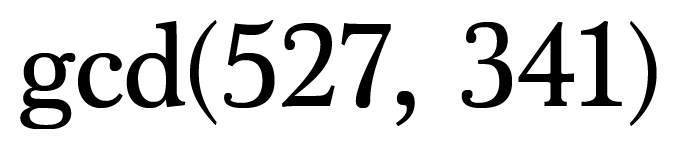The Clever Little Extended Euclidean Algorithm

Today we’re going to take a very quick look at the extended Euclidean Algorithm. If you need a refresher on the standard Euclidean Algorithm, check out this post or this visual explanation.

Alright, let’s jump in!Notation for finding the Greatest Common Divisor (gcd) of 527 and 341

Let’s start by finding the greatest common divisor of 527 and 341 using the standard Euclidean algorithm as a warm-up:

The standard algorithm is succinct and straightforward and will serve as a nice little guide for implementing the extended algorithm.

For the Extended Euclidean Algorithm we’ll take the third equation (in blue), subtract 155(1) from both sides, and do a little rearranging to make an equivalent equation where 31 is isolated.

Next we’ll replace 155 with 341–186(1), which can be found by solving the second equation for 155, giving us the following:

Now let’s clean this up. Make sure to distribute the negative sign through the parenthesis and replace 186 + 186 with 186•2.

As you may notice, we’re simply working our way backwards through the standard algorithm. We’ll need to run through this process one more time to yield an equation involving 527 and 341, which is ultimately our goal.

To do this replace 186 with 527 – 341(1), which comes from the first equation when we solve for 186.

Again simplify the equation by distributing the 2 and combining into groups of 527 and 341.

We have now expressed 31 as a linear combination of 527 and 341. Pretty cool, huh?

Furthermore the number on the opposite side of the linear combination is the greatest common divisor. So in this case 31 is the greatest common divisor of 527 and 341.

The Extended Euclidean Algorithm is handy because it yields Bezout’s Identity as well as highlights the gcd.